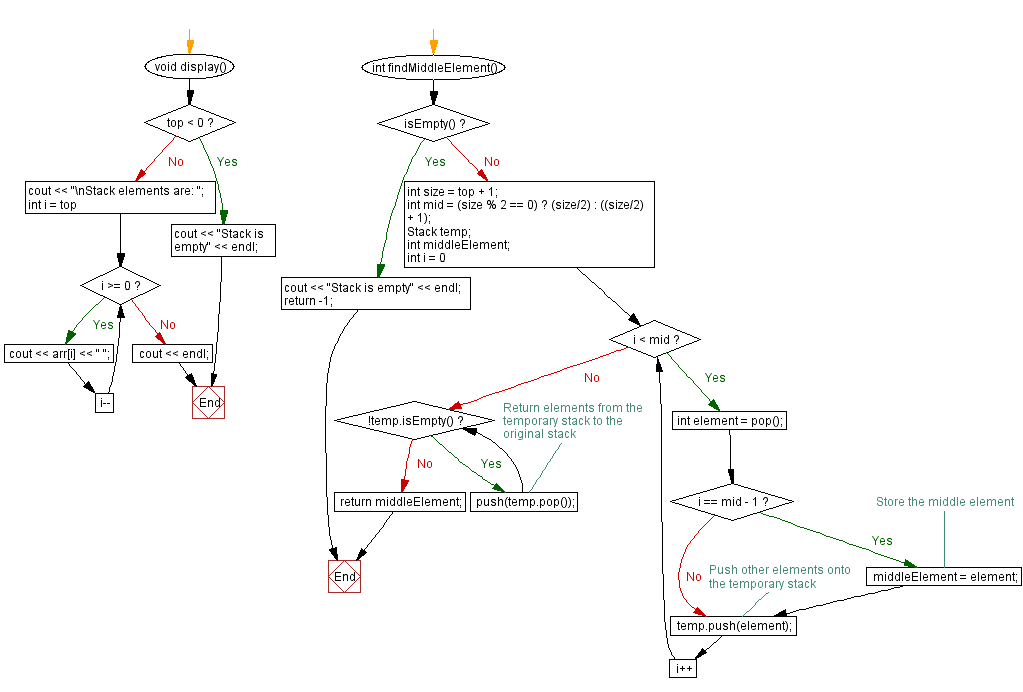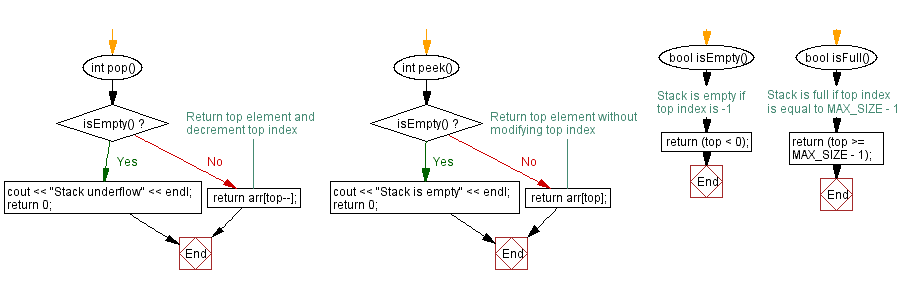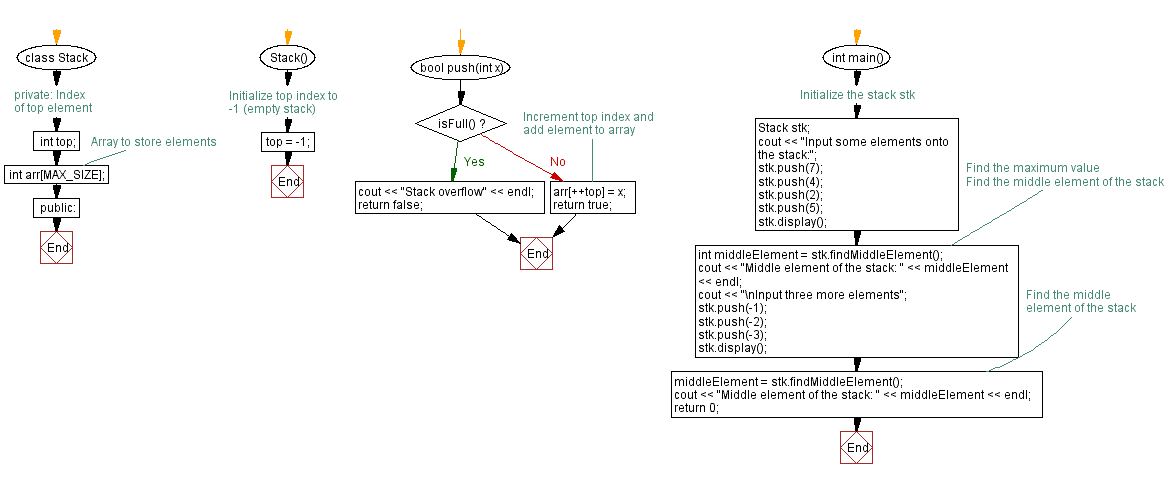﻿ C++ Find the middle element of a stack (using an array)

# C++ Stack Exercises: Find the middle element of a stack (using an array)

## C++ Stack: Exercise-8 with Solution

Write a C++ program to find the middle element of a stack (using an array).

If the number of elements (size) in the stack is odd, return the element at position (size/2) + 1.
If the number of elements is even, return the element at position size/2.

Test Data:
Input some elements onto the stack:
Stack elements are: 5 2 4 7
Middle element of the stack: 2

Sample Solution:

C++ Code:

``````#include <iostream>

using namespace std;
#define MAX_SIZE 15 // Maximum size of stack

class Stack {
private:
int top; // Index of top element
int arr[MAX_SIZE]; // Array to store elements
public:
Stack() {
top = -1; // Initialize top index to -1 (empty stack)
}

bool push(int x) {
if (isFull()) {
cout << "Stack overflow" << endl;
return false;
}
// Increment top index and add element to array
arr[++top] = x;
return true;
}

int pop() {
if (isEmpty()) {
cout << "Stack underflow" << endl;
return 0;
}
return arr[top--];
}

int peek() {
if (isEmpty()) {
cout << "Stack is empty" << endl;
return 0;
}
return arr[top];
}

bool isEmpty() {
// Stack is empty if top index is -1
return (top < 0);
}

bool isFull() {
// Stack is full if top index is equal to MAX_SIZE - 1
return (top >= MAX_SIZE - 1);
}

void display() {
if (top < 0) {
cout << "Stack is empty" << endl;
return;
}
cout << "\nStack elements are: ";
for (int i = top; i >= 0; i--)
cout << arr[i] << " ";
cout << endl;
}

int findMiddleElement() {
if (isEmpty()) {
cout << "Stack is empty" << endl;
return -1;
}

int size = top + 1;
int mid = (size % 2 == 0) ? (size/2) : ((size/2) + 1);

Stack temp;
int middleElement;
for (int i = 0; i < mid; i++) {
int element = pop();
if (i == mid - 1) {
// Store the middle element
middleElement = element;
}
// Push other elements onto the temporary stack
temp.push(element);
}
while (!temp.isEmpty()) {
// Return elements from the temporary stack to the original stack
push(temp.pop());
}
return middleElement;
}
};
int main() {
//Initialize the stack stk
Stack stk;
cout << "Input some elements onto the stack:";
stk.push(7);
stk.push(4);
stk.push(2);
stk.push(5);
stk.display();
// Find the maximum value
int middleElement = stk.findMiddleElement(); // Find the middle element of the stack
cout << "Middle element of the stack: " << middleElement << endl;
cout << "\nInput three more elements";
stk.push(-1);
stk.push(-2);
stk.push(-3);
stk.display();
middleElement = stk.findMiddleElement(); // Find the middle element of the stack
cout << "Middle element of the stack: " << middleElement << endl;
return 0;
}
``````

Sample Output:

```Input some elements onto the stack:
Stack elements are: 5 2 4 7
Middle element of the stack: 2

Input three more elements
Stack elements are: -3 -2 -1 5 2 4 7
Middle element of the stack: 5
```

Flowchart:CPP Code Editor: